# 8 探索性資料分析

## 8.1 事前準備

1. 構思關於這筆資料的問題。

2. 透過視覺化、轉換和建模，試著尋找答案。

3. 經由所得的結果，重整原先的問題，或者構思、增加新的問題。

## 8.2 問題

Far better an approximate answer to the right question, which is often vague, than an exact answer to the wrong question, which can always be made precise.

—John Tukey

1. What type of variation occurs within my variables?

2. What type of covariation occurs between my variables?

## 8.3 Variation

Variation 即每次測量變數值所得的趨勢（tendency）。每個變數都有自己的 variation，而要看出 variation，最好的方法是視覺化變數值的分配。

### 8.3.1 視覺化分配

ggplot(data = diamonds) +
geom_bar(mapping = aes(x = cut))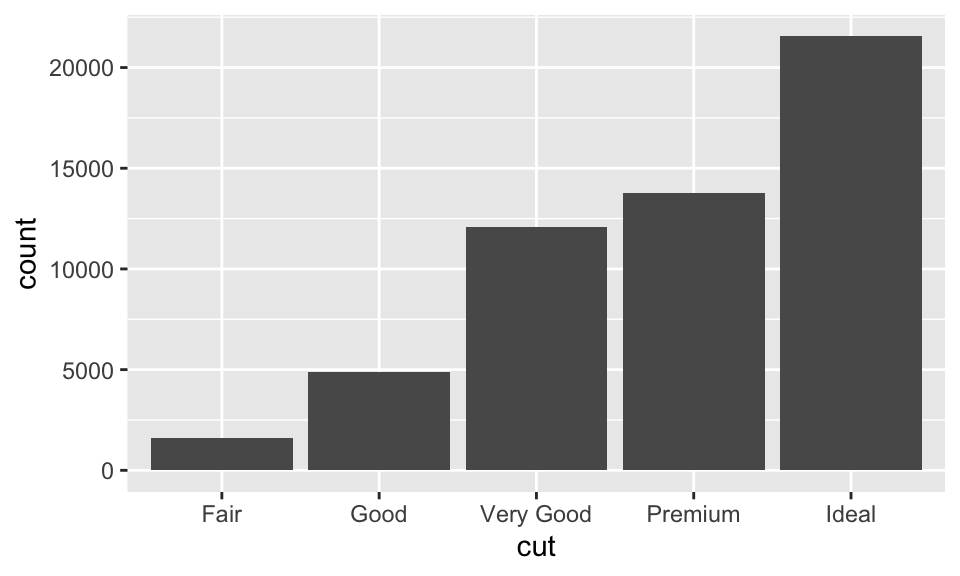diamonds %>%
count(cut)
## # A tibble: 5 × 2
##   cut           n
##   <ord>     <int>
## 1 Fair       1610
## 2 Good       4906
## 3 Very Good 12082
## 5 Ideal     21551

# binwidth 引數即直方圖的直方寬度
ggplot(data = diamonds) +
geom_histogram(mapping = aes(x = carat), binwidth = 0.5)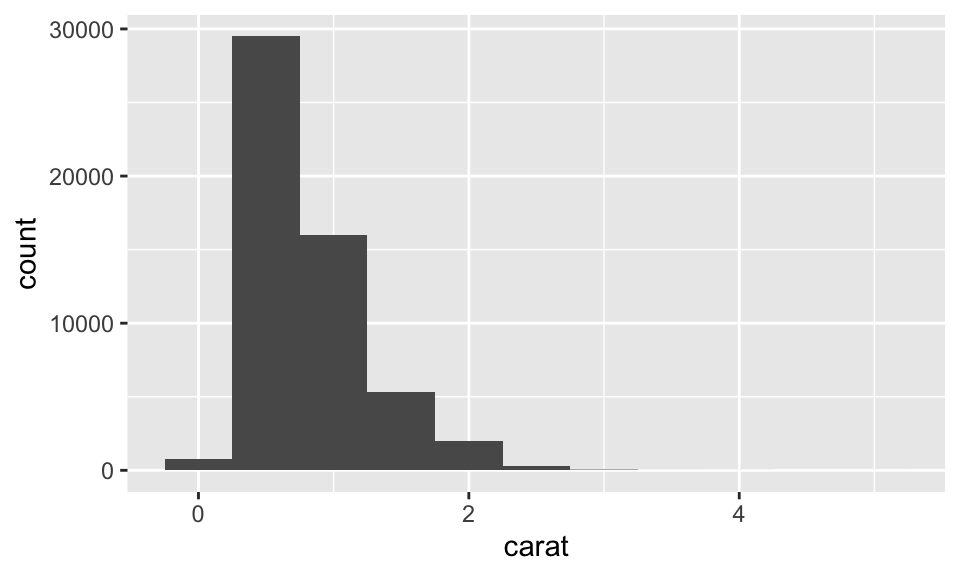diamonds %>%
count(cut_width(carat, 0.5))
## # A tibble: 11 × 2
##    cut_width(carat, 0.5)     n
##    <fct>                   <int>
##  1 [-0.25,0.25]              785
##  2 (0.25,0.75]             29498
##  3 (0.75,1.25]             15977
##  4 (1.25,1.75]              5313
##  5 (1.75,2.25]              2002
##  6 (2.25,2.75]               322
##  7 (2.75,3.25]                32
##  8 (3.25,3.75]                 5
##  9 (3.75,4.25]                 4
## 10 (4.25,4.75]                 1
## 11 (4.75,5.25]                 1

ggplot(data = diamonds, mapping = aes(x = carat, color = cut)) +
geom_freqpoly(binwidth = 0.1)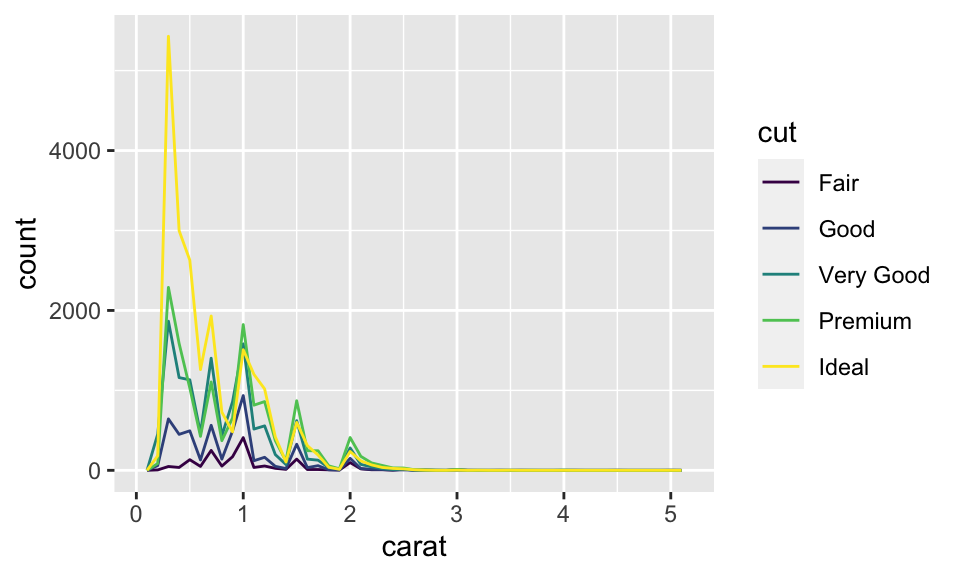### 8.3.2 代表性的變數值

ggplot(data = diamonds, mapping = aes(x = carat)) +
geom_histogram(binwidth = 0.01)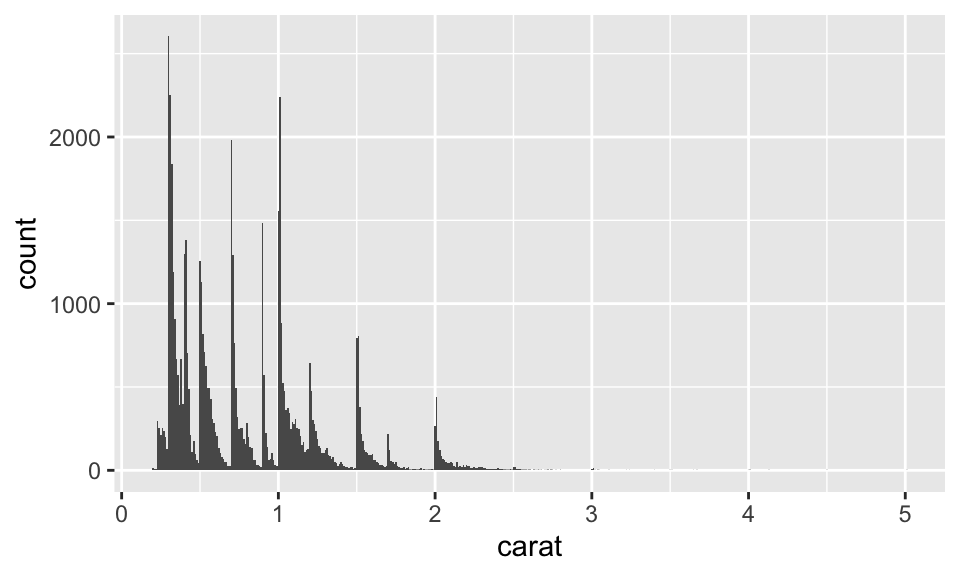1. 每個 subgroup 中的觀察值與其他 subgroups 之間有何相異或相似？

2. 如何描述或解釋群集？

3. 為何群集的外觀可能產生誤導？

### 8.3.3 不尋常的變數值

Outliers 即不尋常的變數值，可能源自資料輸入錯誤，也可能是一些不一樣的東西。

ggplot(diamonds) +
geom_histogram(mapping = aes(x = y), binwidth = 0.5) +
coord_cartesian(ylim = c(0, 50))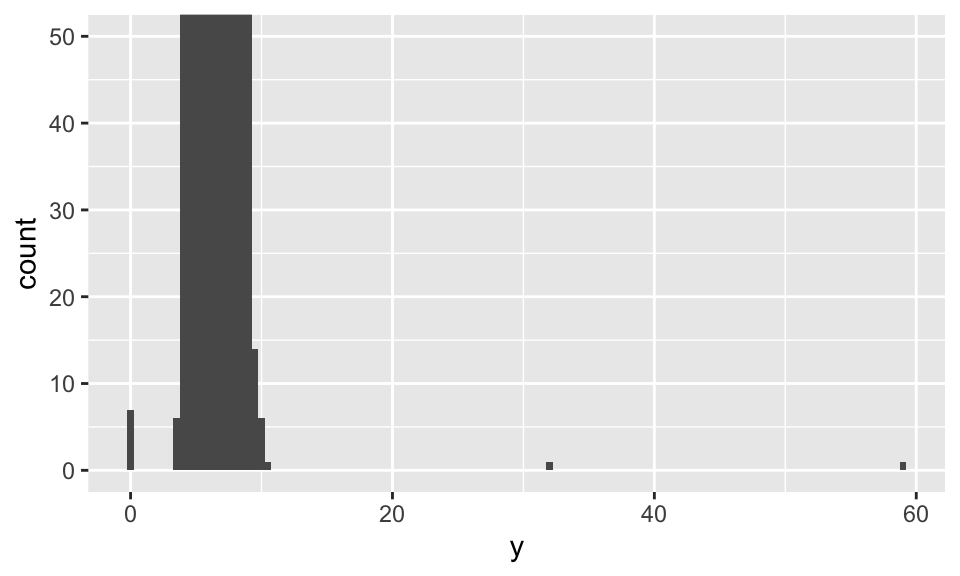unusual <- diamonds %>%
filter(y < 3 | y > 20) %>%
arrange(y)

unusual
## # A tibble: 9 × 10
##   carat cut       color clarity depth table price     x     y     z
##   <dbl> <ord>     <ord> <ord>   <dbl> <dbl> <int> <dbl> <dbl> <dbl>
## 1  1    Very Good H     VS2      63.3    53  5139  0      0    0
## 2  1.14 Fair      G     VS1      57.5    67  6381  0      0    0
## 3  1.56 Ideal     G     VS2      62.2    54 12800  0      0    0
## 4  1.2  Premium   D     VVS1     62.1    59 15686  0      0    0
## 5  2.25 Premium   H     SI2      62.8    59 18034  0      0    0
## 6  0.71 Good      F     SI2      64.1    60  2130  0      0    0
## 7  0.71 Good      F     SI2      64.1    60  2130  0      0    0
## 8  0.51 Ideal     E     VS1      61.8    55  2075  5.15  31.8  5.12
## 9  2    Premium   H     SI2      58.9    57 12210  8.09  58.9  8.06

## 8.4 Missing Value

### 8.4.1 替換掉 Outliers

1. 丟棄有奇怪的變數值的觀察值。可是，其中一個變數輸入錯誤不代表其他變數就也輸入錯誤。而且如果資料品質不良，可能最後什麼都不剩。
# 丟棄有奇怪的變數值的觀察
diamonds2 <- diamonds %>%
filter(between(y, 3, 20))
1. （推薦）把 outliers 的變數值換成 NA。我們可以使用 mutate()ifelse()，如：
# 把 outliers 的變數值換成 NA
diamonds2 <- diamonds %>%
mutate(y = ifelse(y < 3 | y > 20, NA, y))
# 這樣畫出來的散佈圖就不會是 y 的 outliers
ggplot(data = diamonds2, mapping = aes(x = x, y = y)) +
geom_point(na.rm = TRUE)  # 注意：設定 na.rm = TRUE，忽略掉 NA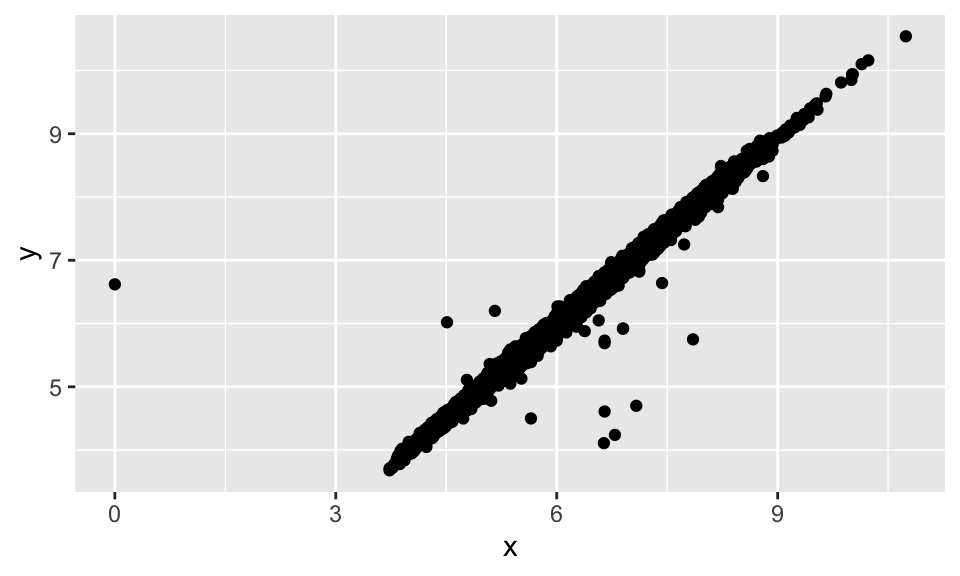### 8.4.2 比較 NA 與否

nycflights13::flights %>%
mutate(
cancelled = is.na(dep_time),
sched_hour = sched_dep_time %/% 100,
sched_min = sched_dep_time %% 100,
sched_dep_time = sched_hour + sched_min / 60) %>%
ggplot(mapping = aes(sched_dep_time)) +
geom_freqpoly(mapping = aes(color = cancelled), binwidth = 1/4)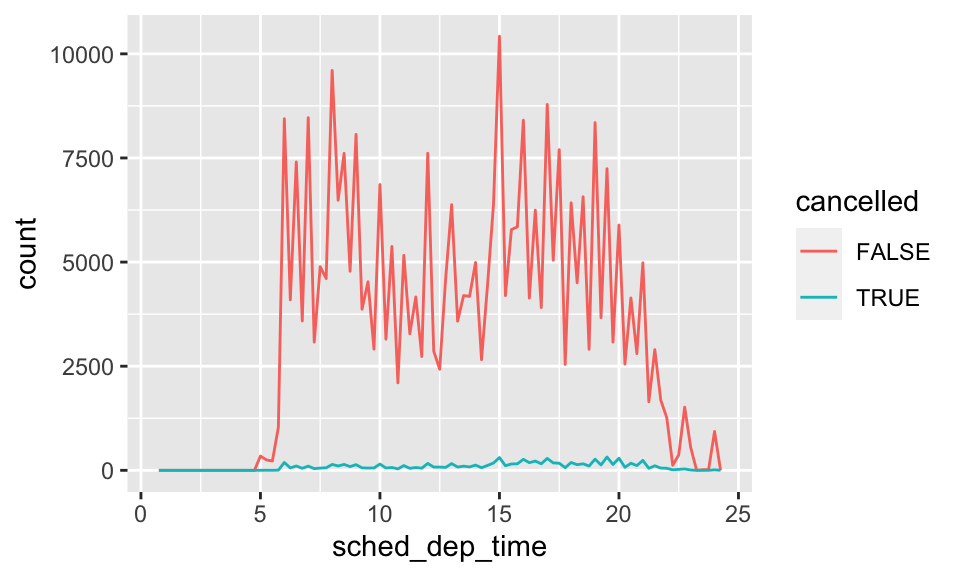## 8.5 Covariation

Covariation 即兩個或多個變數變動的關係。想要發覺 covariation，最好的辦法就是視覺化它。但如何視覺化則牽涉到變數型態的問題。

### 8.5.1 類別與連續變數

ggplot(data = diamonds, mapping = aes(x = price)) +
geom_freqpoly(mapping = aes(color = cut), binwidth = 500)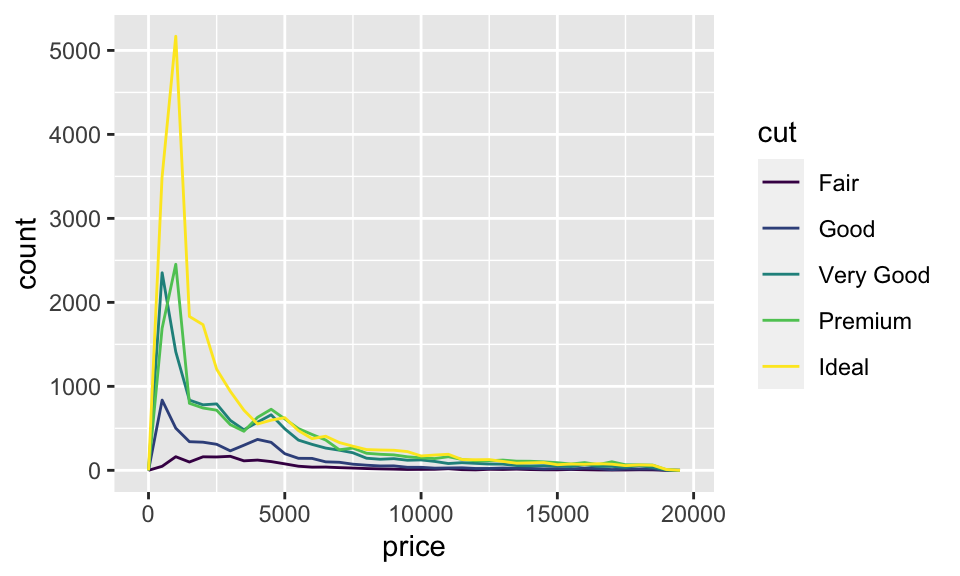ggplot(data = diamonds, mapping = aes(x = price, y = ..density..)) +
geom_freqpoly(mapping = aes(color = cut), binwidth = 500)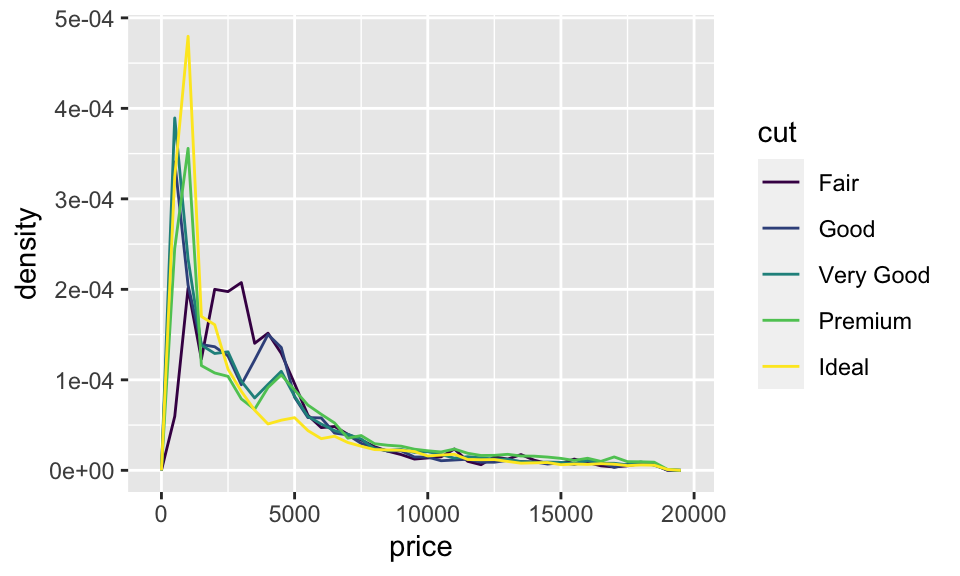ggplot(data = diamonds, mapping = aes(x = cut, y = price)) +
geom_boxplot()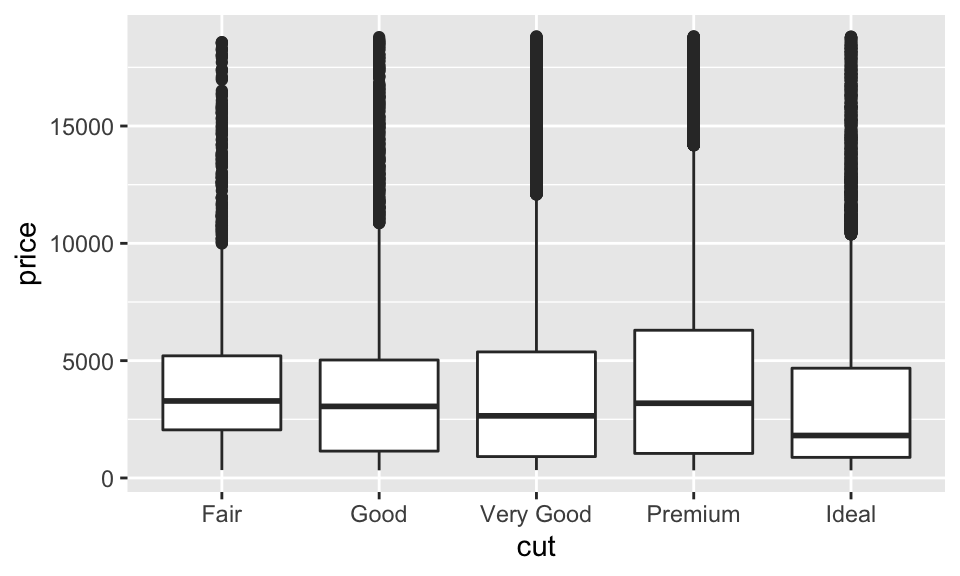ggplot(data = diamonds, mapping = aes(x = cut, y = carat)) +
geom_boxplot()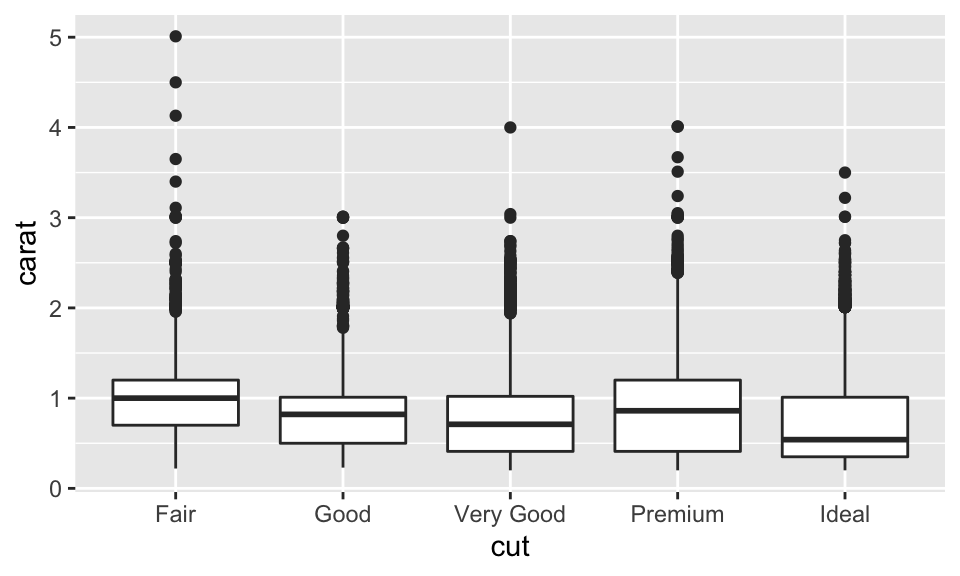ggplot(data = mpg, mapping = aes(x = class, y = hwy)) +
geom_boxplot()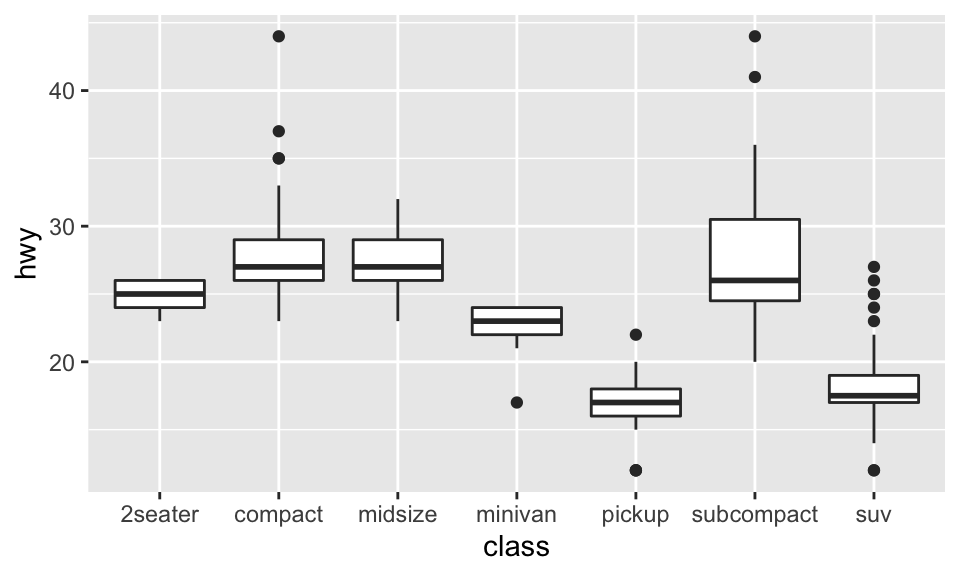reorder(x, X, FUN = mean, ...,
order = is.ordered(x))

ggplot(data = mpg) +
geom_boxplot(mapping = aes(
x = reorder(class, hwy, FUN = median),
y = hwy
))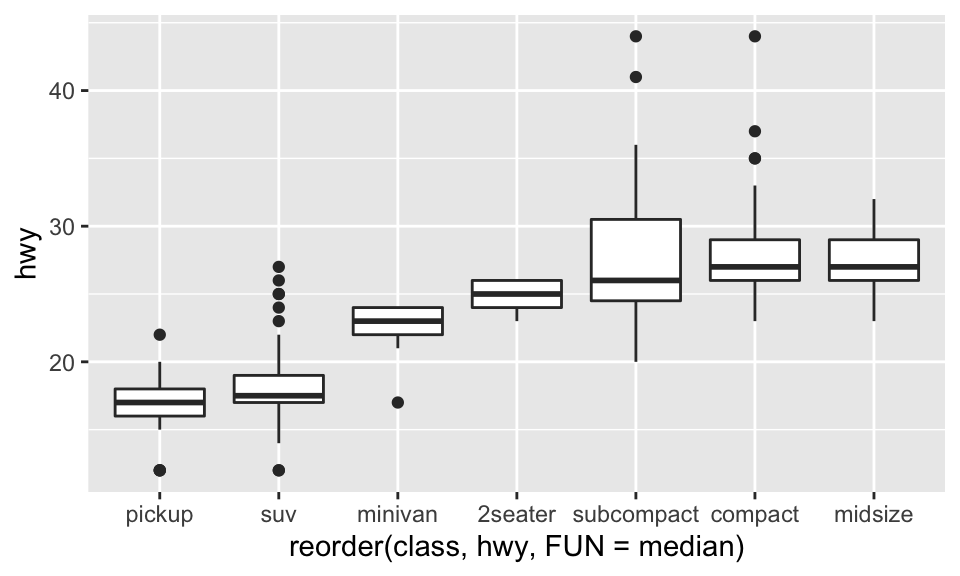ggplot(data = mpg) +
geom_boxplot(mapping = aes(
x = reorder(class, hwy, FUN = median),
y = hwy
)) + coord_flip()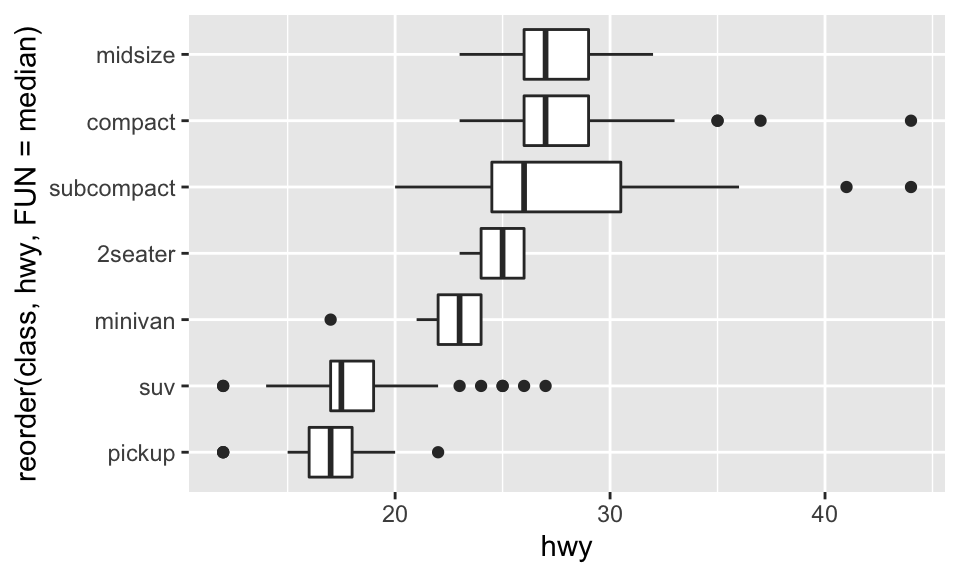### 8.5.2 兩個類別變數

ggplot(data = diamonds) +
geom_count(mapping = aes(x = cut, y = color))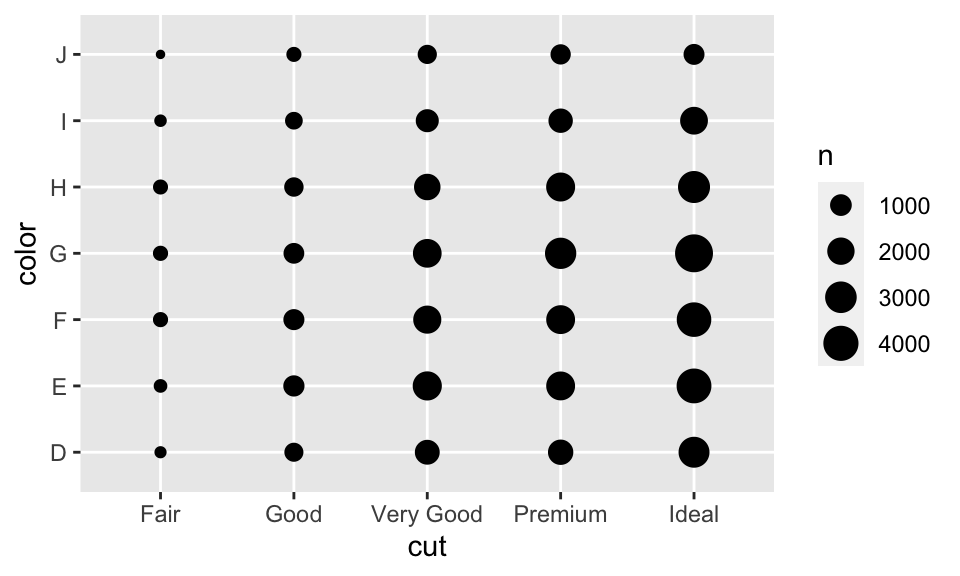diamonds %>%
count(color, cut)
## # A tibble: 35 × 3
##    color cut           n
##    <ord> <ord>     <int>
##  1 D     Fair        163
##  2 D     Good        662
##  3 D     Very Good  1513
##  5 D     Ideal      2834
##  6 E     Fair        224
##  7 E     Good        933
##  8 E     Very Good  2400
## 10 E     Ideal      3903
## # … with 25 more rows

diamonds %>%
count(color, cut) %>%
ggplot(mapping = aes(x = color, y = cut)) +
geom_tile(mapping = aes(fill = n))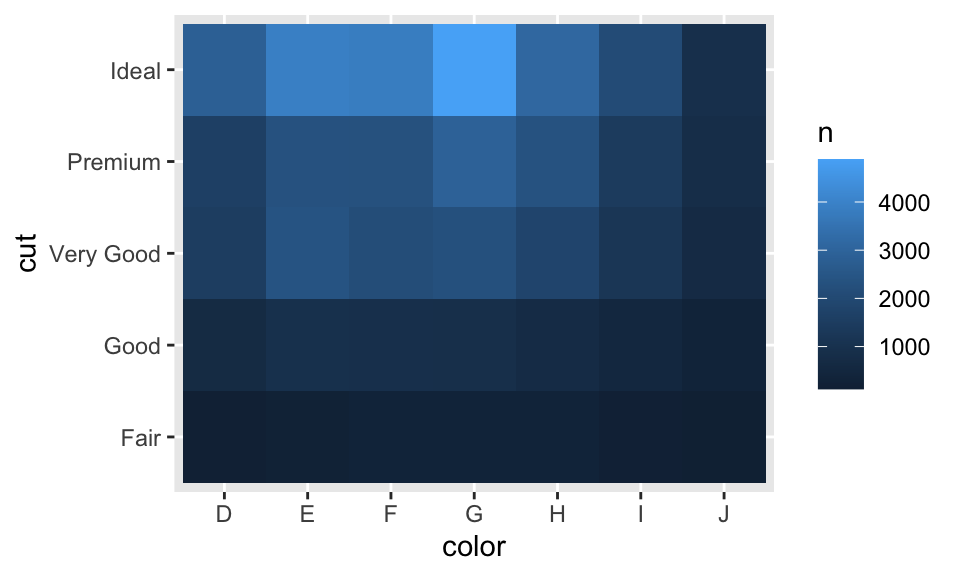### 8.5.3 兩個連續變數

ggplot(data = diamonds) +
geom_point(mapping = aes(x = carat, y = price))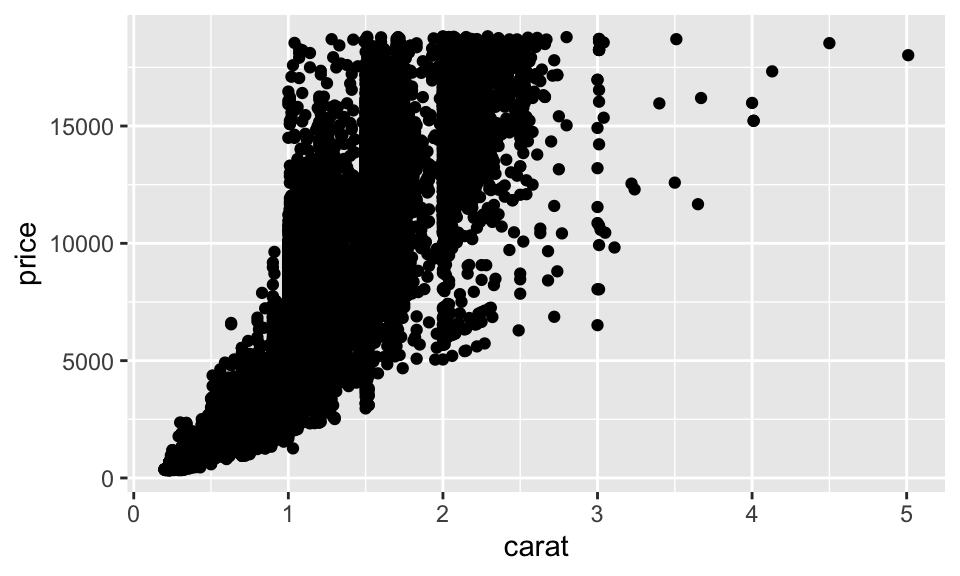ggplot(data = diamonds) +
geom_point(mapping = aes(x = carat, y = price),
alpha = 1/100)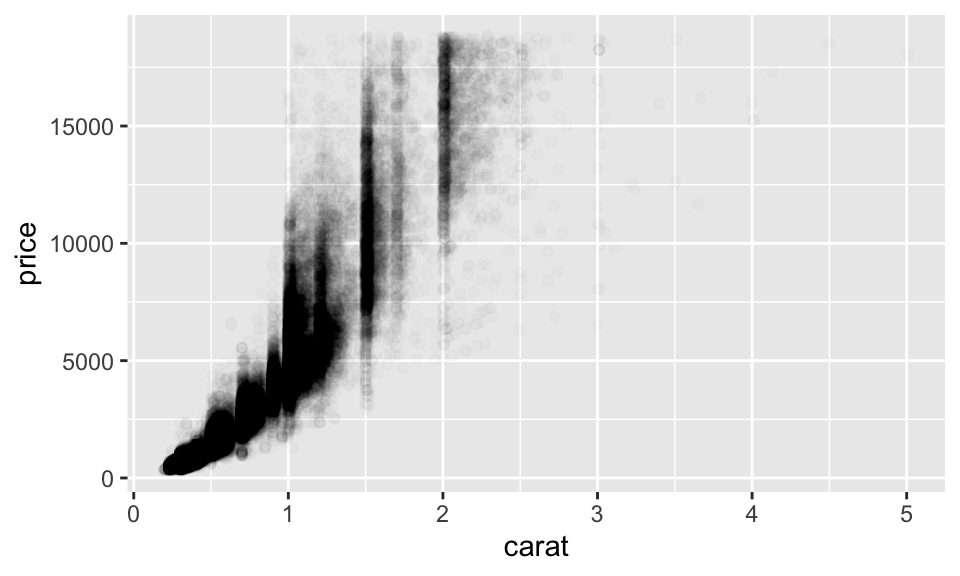geom_bin2d()geom_hex() 所繪製出來的圖形樣式相似，都是有二維的 bins，而使用 fill 來表示數量，差別在 geom_bin2d() 繪製出來的是矩形，而 geom_hex() 繪製出來的是六邊形，如：

ggplot(data = diamonds) +
geom_bin2d(mapping = aes(x = carat, y = price))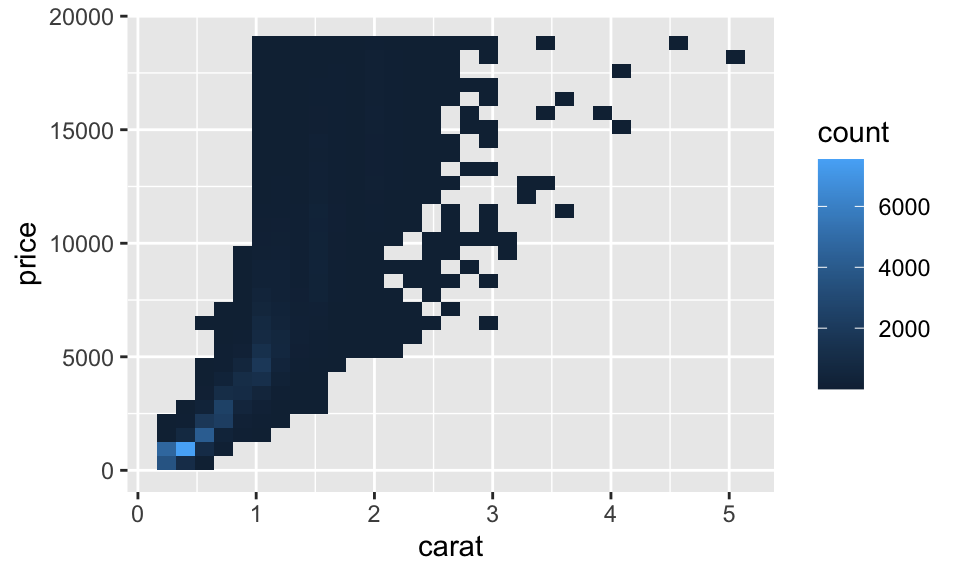# install.packages("hexbin")
library(hexbin)
ggplot(data = diamonds) +
geom_hex(mapping = aes(x = carat, y = price))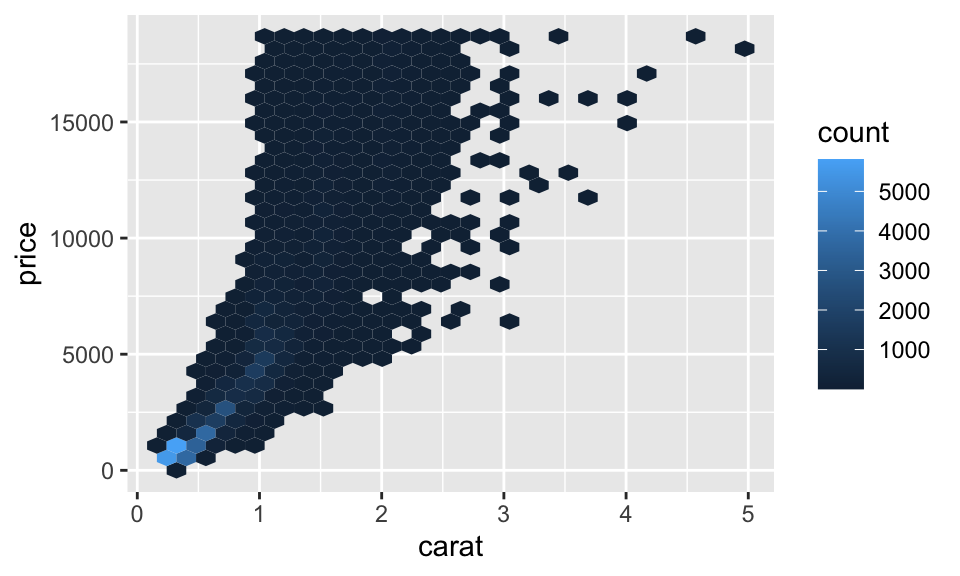ggplot(data = diamonds, mapping = aes(x = carat, y = price)) +
geom_boxplot(mapping = aes(group = cut_width(carat, 0.3)))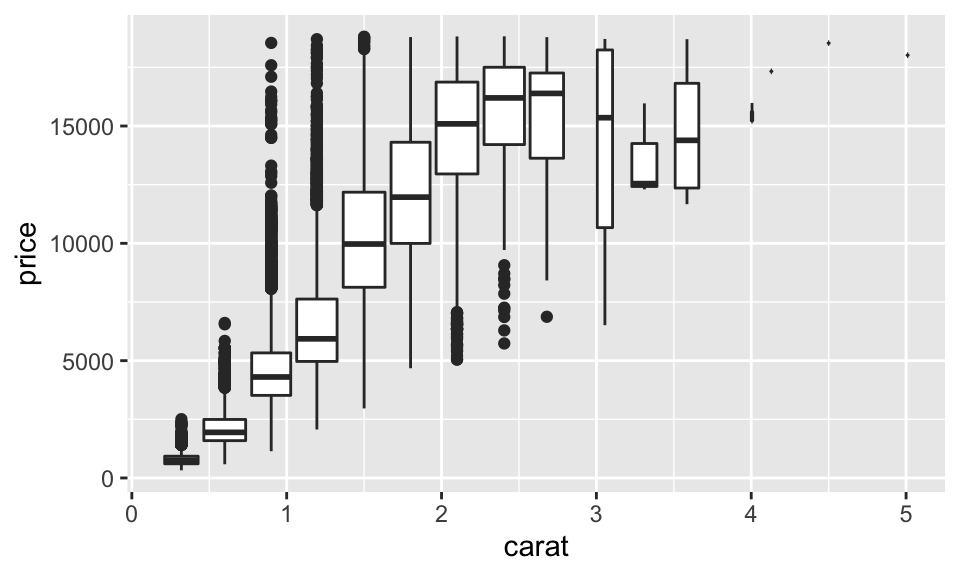ggplot(data = diamonds, mapping = aes(x = carat, y = price)) +
geom_boxplot(mapping = aes(group = cut_width(carat, 0.3)), varwidth = TRUE)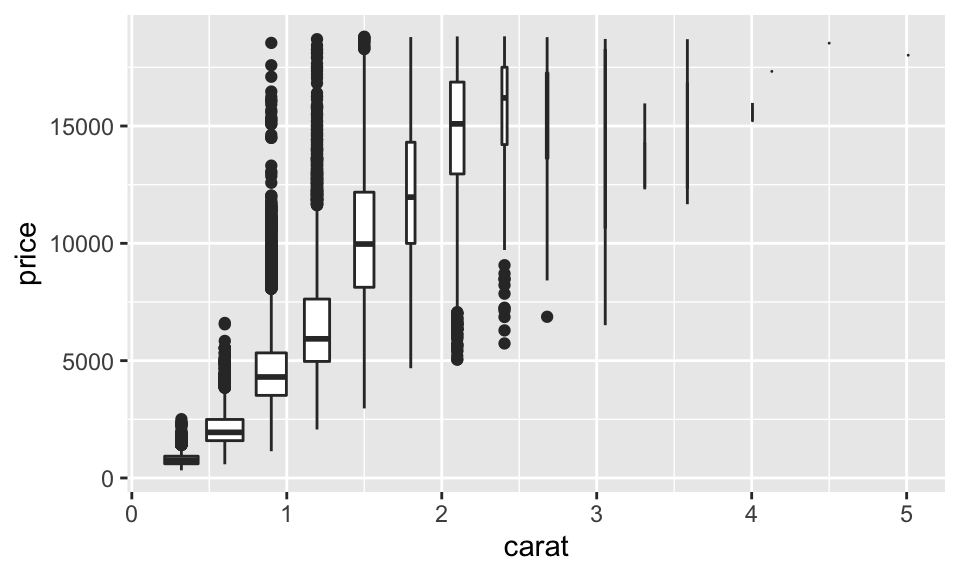ggplot(data = diamonds, mapping = aes(x = carat, y = price)) +
geom_boxplot(mapping = aes(group = cut_number(carat, 20)))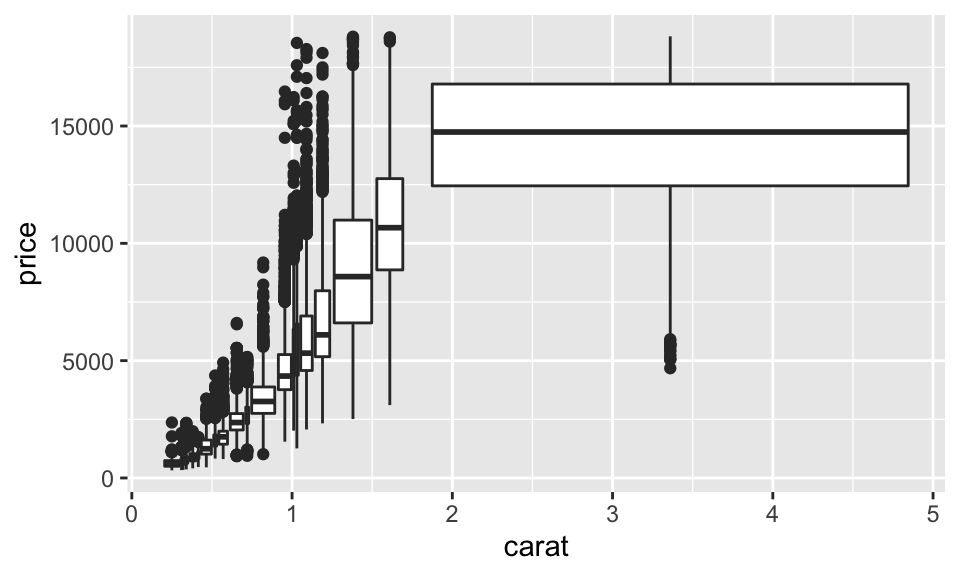## 8.6 模式與模型

ggplot(data = faithful) +
geom_point(mapping = aes(x = eruptions, y = waiting))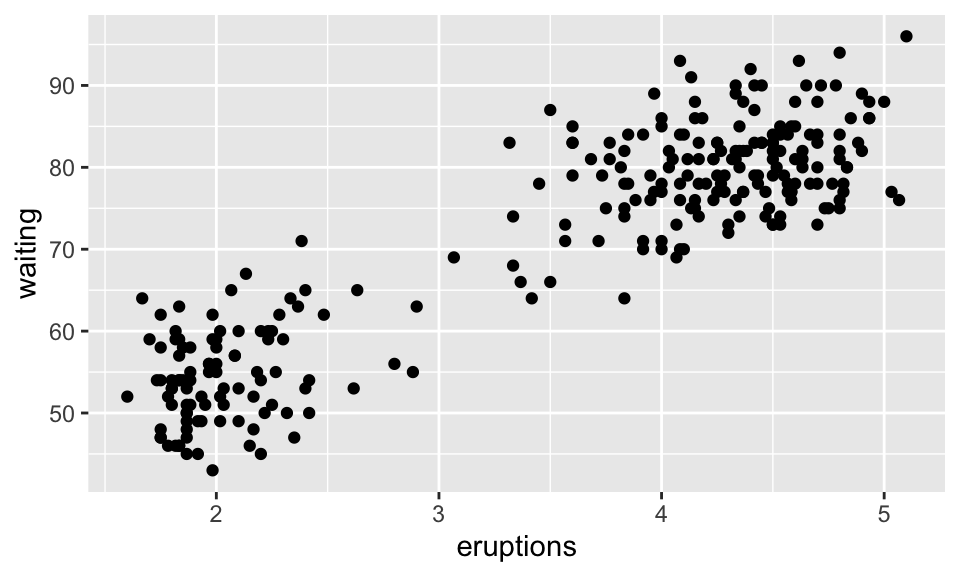library(modelr)
mod <- lm(log(price) ~ log(carat), data = diamonds)

diamonds2 <- diamonds %>%
mutate(resid = exp(resid))

ggplot(data = diamonds2) +
geom_point(mapping = aes(x = carat, y = resid))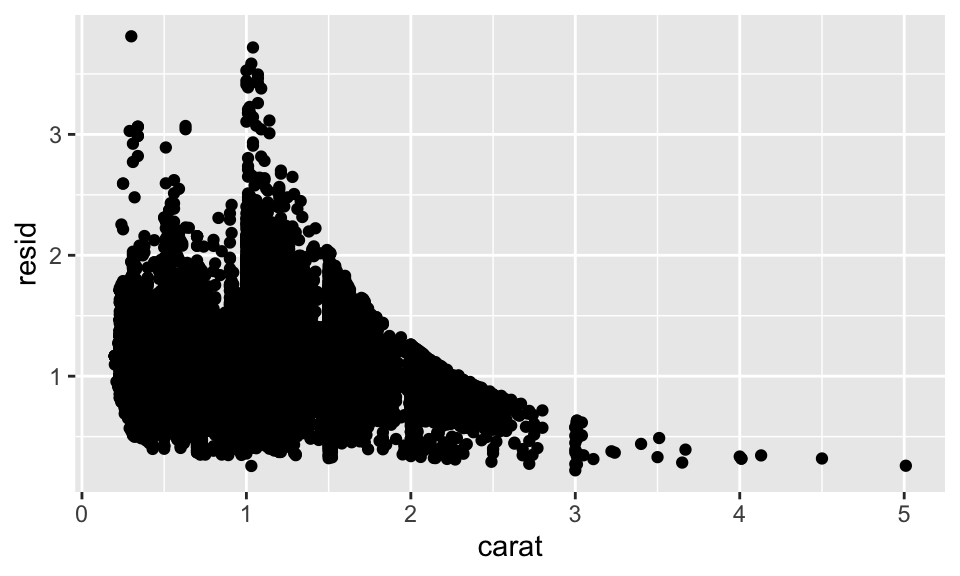ggplot(data = diamonds2) +
geom_boxplot(mapping = aes(x = cut, y = resid))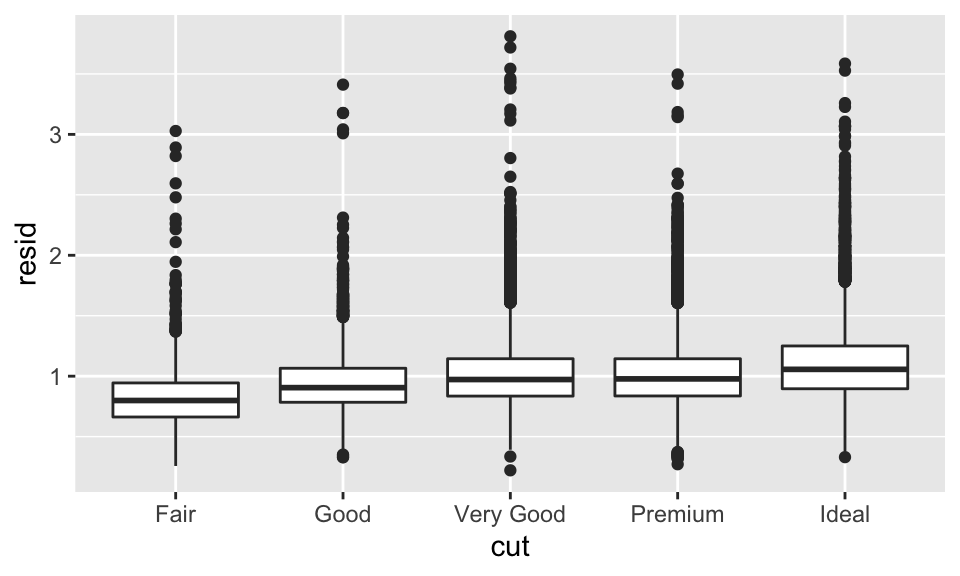### 參考文獻

Wickham, Hadley, and Garrett Grolemund. 2016. R for Data Science. First Edition. O’Reilly Media, Inc.